cancel
Showing results for
Did you mean:New Member

## Charting an Electrocardiogram from a single value

Hi All,

I'm trying to chart an Electrocardiogram from a  value that contains 2500 values separated by a space. My dataset looks like this: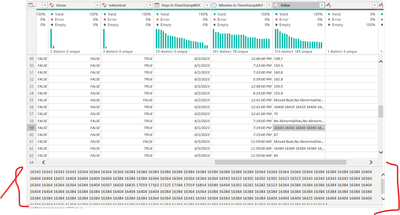I'm trying to create a chart with the numbers in the cell and a fixed X-axis value (let's say 2,000). My chart should look like this: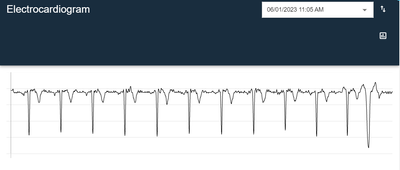I have tried using split.text and Generate series in DAX, but since this is medical data, I want to make sure that if for some reason one data point doesn't have 2,500 points, that I don't mess up the whole thing. What could be the best way to create this graph?

Thanks!

1 ACCEPTED SOLUTIONCommunity Support

According to your description, here's my solution.

Sample: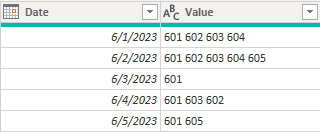1. Replace value in Power Query. For the Value column, replace space to "|".2.Create a calculated column.

``````PathNo =
PATHLENGTH ( [Value] )
``````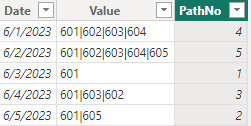3.Create a new table.

``````Table 2 =
GENERATESERIES ( 1, MAX ( 'Table'[PathNo] ), 1 )
``````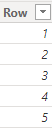4.Create a measure.

``````Measure =
CONVERT (
PATHITEM ( SELECTEDVALUE ( 'Table'[Value] ), MAX ( 'Table 2'[Row] ) ),
INTEGER
)
``````

Put Row column in X-axis and measure in Y-axis, get the correct result.I attach my sample below for your reference.

Best Regards,
Community Support Team _ kalyj

If this post helps, then please consider Accept it as the solution to help the other members find it more quickly.

2 REPLIES 2Community Support

According to your description, here's my solution.

Sample:1. Replace value in Power Query. For the Value column, replace space to "|".2.Create a calculated column.

``````PathNo =
PATHLENGTH ( [Value] )
``````3.Create a new table.

``````Table 2 =
GENERATESERIES ( 1, MAX ( 'Table'[PathNo] ), 1 )
``````4.Create a measure.

``````Measure =
CONVERT (
PATHITEM ( SELECTEDVALUE ( 'Table'[Value] ), MAX ( 'Table 2'[Row] ) ),
INTEGER
)
``````

Put Row column in X-axis and measure in Y-axis, get the correct result.I attach my sample below for your reference.

Best Regards,
Community Support Team _ kalyj

If this post helps, then please consider Accept it as the solution to help the other members find it more quickly.New Member

Thank you